# Karnaugh Map Solver

To use the Karnaugh map calculator, select the type of calculation i.e SoP or PoS, name the output (optional), enter the “Input names”, and click calculate button

Give Us Feedback

## Karnaugh Map Solver

This K-Map solver performs two tasks. Firstly, it makes the k-map, and secondly, it calculates the Sum of the products or Product of the sums of boolean expressions.

It allows you to name the output and use the “don’t cares”. The Karnaugh map solver provides three different solution representations namely:

1. VHDL
2. Generic
3. Verilog

## What is a karnaugh map?

It is a table, very similar to the truth table, that represents boolean expressions pictorially. By using the Karnaugh map, solving the boolean expressions and their sum and products becomes relatively easy.

### The formula of the karnaugh map:

The Karnaugh map is made using the exponent operation. Its formula is 2n. The letter “n” represents the number of inputs or variables. The resultant number tells the number of cells or squares that are required in the map.

For example, if h(a,b,c) then the k-map will consist of 8 cells because 2 raised to power 3 (`no. of variables`) is 8.

### Row and column number:

Depending on the number of variables, each row and column has a number. If there are only two variables, the number goes like 0,1 for both rows and columns

For three and four variables, see the maps coming ahead.

### Cell number:

Each cell of the k-map has its own number and these numbers are fixed. They cannot be changed. This number is assigned to the cell after multiplying the row and column binary number and converting the answer from binary to decimal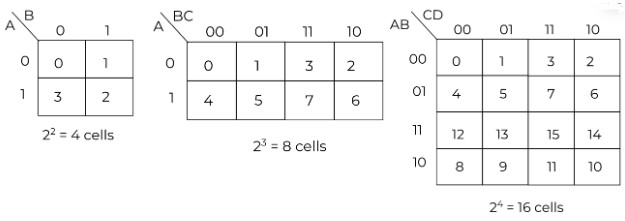### Making of groups:

For making groups, write “1” at the place of every cell number given in the question. Then make groups of 2, 4, or 8 cells. It must be a power of 2. Always aim for one bigger group instead of making two or three smaller groups.

Note that groups should always be either horizontal or vertical, never diagonal. One group can overlap with another group.

Don’t cares are marked with “x”. They help to complete a group and can be treated as “1” or “0” depending on the situation.

### How to make a karnaugh map?

The method of making a Karnaugh map is more comprehensible if you look at an example.

Example:

Make the k-map for the following expression.

`g(A,B,C) = (0,3,5,7)`

Solution:

Step 1: Find the number of cells.

There are 3 variables so 23 is 8.

Step 2: Make the cells and write “1” at the place of the numbers.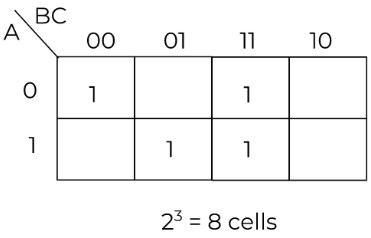Step 3: Make groups.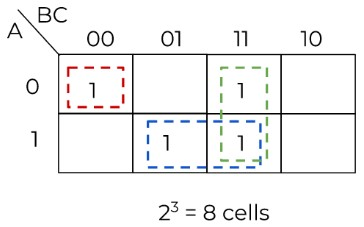One singleton and two pairs are formed.

## SoP and PoS of boolean expressions:

For the `sum of the products`, 0 means the variable has a complement. Add “Dot(.)” between variables of a group and add “sum(+)” between the groups.

For the `products of the sum`, 1 means the variable has a complement. Add “sum (+)” between the variables of a group and add “dot(.)” between groups.

Cancel all the pairs of 1 and 0

### Example: (Sum of products)

For the following expression, find the sum of products.

`h (A, B, C, D) = (9,10,2,1,11,5)`

Don't care = (0)

Solution:

Step 1: Draw the k-map.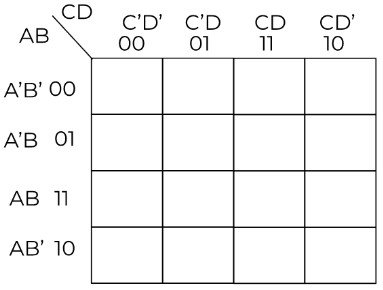Step 2: Write 1 for every number given in the question and x for don’t care.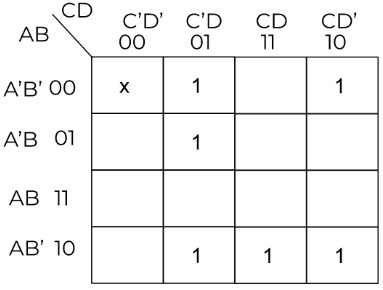Step 3: Make the groups and write their binary value in a table.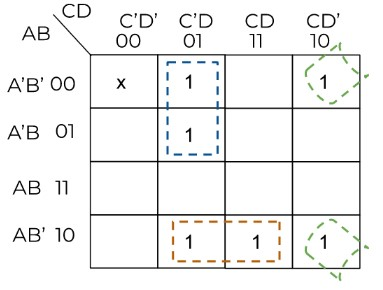Three pairs are formed.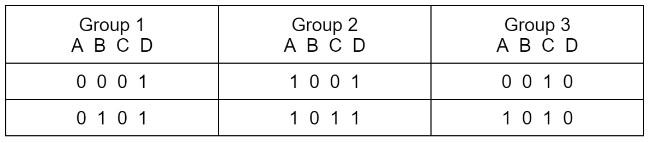Cancelation: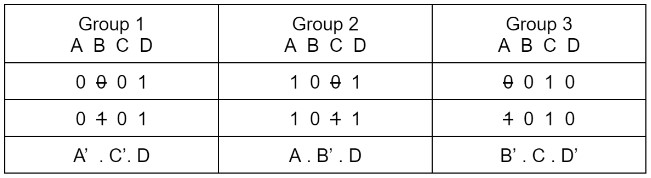Step 4: Write the sum of products.

`H =    (A’  . C’. D) + (A . B’ . D) + (B’ . C . D’)`

### Example: (Product of Sums)

For the following expression, find the product of sums.

`h (A,B,C,D) = (9,10,2,1,11,5)`

Don't care = (0)

Solution:

Step 1: Draw the k-map.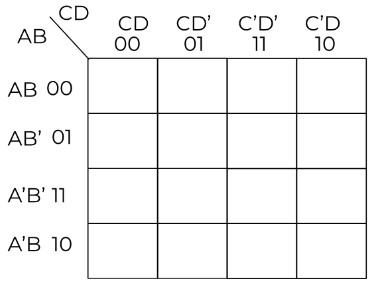Step 2: Write 0 in every cell except the numbers given in the question and x for don’t care.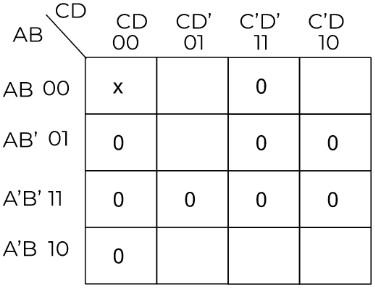Step 3: Make the groups and write their binary value in a table.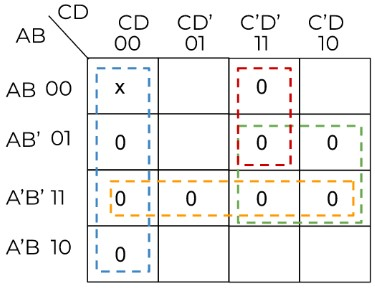Four quads and one pair are formed.

Cancelation: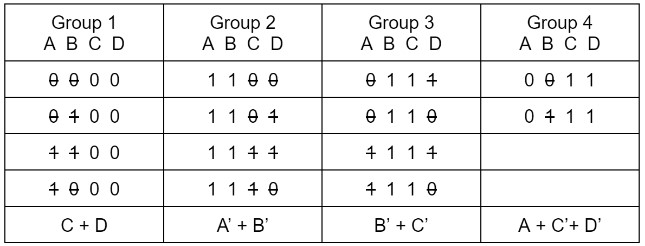Step 4: Write the product of sums.

`H =    (C + D) . (A’ + B’) . (B’ + C’) . (A + C’+ D’)`

### Math Tools